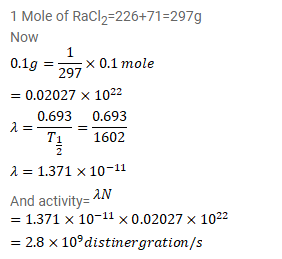# The half-life of 226Ra is 1602 y.

Question:

The half-life of ${ }^{226} \mathrm{Ra}$ is $1602 \mathrm{y}$. Calculate the activity of $0.1 \mathrm{~g}$ of $\mathrm{RaCl}_{2}$ in which all the radium is in the form of ${ }^{220} \mathrm{Ra}$. Taken atomic weight of Ra to be $226 \mathrm{~g} / \mathrm{mol}$ and that of $C l$ to be $35.5 \mathrm{~g} / \mathrm{mol}$.

Solution: